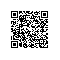# [译]Functor 与 Category （软件编写）（第六部分）

<上一篇 | << 返回第一章

“functor” 一词源于范畴学。在范畴学中，一个 functor 代表了两个范畴（category）间的映射。简单说来，一个 范畴 是一系列事物的分组，这里的 “事物” 可以指代一切的值。对于编码来说，一个 functor 通常代表了一个具有 .map() 方法的对象，该方法能够将某一集合映射到另一集合。

fmap :: (a -> b) -> f a -> f b

fmap 接受一个函数参数，该函数接受一个参数 a，并返回一个 b，最终，fmap 完成了从f a 到 f b 的映射。f a 及 f b 可以被读作 “一个 a 的 functor” 和“一个 b 的 functor”，亦即 f a 这个容器容纳了 af b 这个容器容纳了 b

const f = [1, 2, 3];
f.map(double); // [2, 4, 6]

### Functor 定律

1. 同一性（Identity）
2. 组合性（Composition）

### 同一性

const f = [1, 2, 3];
f.map(x => x); // [1, 2, 3]

### 组合性

functor 还必须具有组合性：F.map(x => f(g(x))) == F.map(g).map(f)

• 一个范畴（category）是一个容纳了一系列对象及对象间箭头（->）的集合。
• 箭头只是形式上的描述，实际上，箭头代表了态射（morphismms）。在编程中，态射可以被认为是函数。
• 对于任何被箭头相连接的对象，如 a -> b -> c，必须存在一个 a -> c 的组合。
• 所有的箭头表示都代表了组合（即便这个对象间的组合只是一个同一（identity）箭头：a->c）。所有的对象都存在一个同一箭头，即存在同一态射（a -> a）。

h∘(g∘f) = (h∘g)∘f = h∘g∘f

const F = [1, 2, 3];

F.map(x => f(g(x)));

// 等效于......

F.map(g).map(f);

### 自定义一个 Functor

const Identity = value => ({
map: fn => Identity(fn(value))
});

// trace() 是一个简单的工具函数来帮助审查内容
// 内容
const trace = x => {
console.log(x);
return x;
};

const u = Identity(2);

// 同一性
u.map(trace);             // 2
u.map(x => x).map(trace); // 2

const f = n => n + 1;
const g = n => n * 2;

// 组合性
const r1 = u.map(x => f(g(x)));
const r2 = u.map(g).map(f);

r1.map(trace); // 5
r2.map(trace); // 5

const Identity = value => ({
map: fn => Identity(fn(value)),

valueOf: () => value,
});

const ints = (Identity(2) + Identity(4));
trace(ints); // 6

const hi = (Identity('h') + Identity('i'));
trace(hi); // "hi"

toString: () => Identity(${value}), 代码又有所进步。现在，我们可能也想 functor 能够满足标准的 JavaScript 迭代协议（译注：MDN - 迭代协议）。为此，我们可以为 Identity 添加一个自定义的迭代器：  [Symbol.iterator]: () => { let first = true; return ({ next: () => { if (first) { first = false; return ({ done: false, value }); } return ({ done: true }); } }); }, 现在，我们的 functor 还能这样工作: // [Symbol.iterator] enables standard JS iterations: const arr = [6, 7, ...Identity(8)]; trace(arr); // [6, 7, 8] 假如你想借助 Identity(n) 来返回包含了 n+1n+2 等等的 Identity 数组，这非常容易： const fRange = ( start, end ) => Array.from( {length: end - start + 1}, (x, i) => Identity(i + start) ); 但是，如果你想上面的操作方式能够应用于任何 functor，该怎么办？假如我们规定了每种数据类型对应的实例必须有一个关于其构造函数的引用，那么你可以这样改造之前的逻辑： const fRange = ( start, end ) => Array.from( {length: end - start + 1}, // 将 Identity 变更为 start.constructor (x, i) => start.constructor(i + start) ); const range = fRange(Identity(2), 4); range.map(x => x.map(trace)); // 2, 3, 4 假如你还想知道一个值是否在一个 functor 中，又怎么办？我们可以为 Identity 添加一个静态方法 .is() 来进行检测，另外，我们也顺便添加了一个静态的 .toString() 方法来告知这个 functor 的种类： Object.assign(Identity, { toString: () => 'Identity', is: x => typeof x.map === 'function' }); 现在，我们整合一下上面的代码片: const Identity = value => ({ map: fn => Identity(fn(value)), valueOf: () => value, toString: () => Identity(${value}),

[Symbol.iterator]: () => {
let first = true;
return ({
next: () => {
if (first) {
first = false;
return ({
done: false,
value
});
}
return ({
done: true
});
}
});
},

constructor: Identity
});

Object.assign(Identity, {
toString: () => 'Identity',
is: x => typeof x.map === 'function'
});

### 为什么要使用 functor?

// 创建一个 predicte
const exists = x => (x.valueOf() !== undefined && x.valueOf() !== null);

const ifExists = x => ({
map: fn => exists(x) ? x.map(fn) : x
});

const add1 = n => n + 1;
const double = n => n * 2;

// undefined
ifExists(Identity(undefined)).map(trace);
// null
ifExists(Identity(null)).map(trace);

// 42
ifExists(Identity(20))
.map(double)
.map(trace)
;

const curry = (
f, arr = []
) => (...args) => (
a => a.length === f.length ?
f(...a) :
curry(f, a)
)([...arr, ...args]);

const map = curry((fn, F) => F.map(fn));

const double = n => n * 2;

const mdouble = map(double);
mdouble(Identity(4)).map(trace); // 8

### 总结

functor 是能够对其进行 map 操作的对象。更进一步地，一个 functor 能够将一个范畴映射到另一个范畴。一个 functor 甚至可以将某一范畴映射回相同范畴（例如 endofunctor）。使用钉钉扫一扫加入圈子
+ 订阅